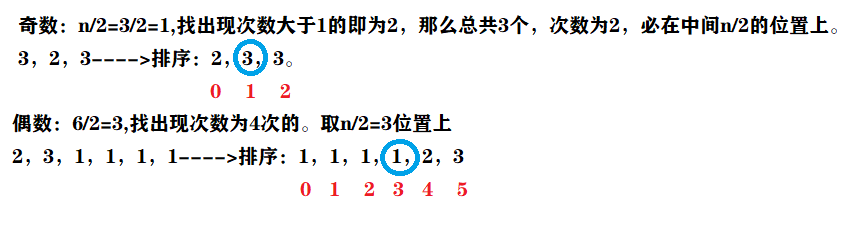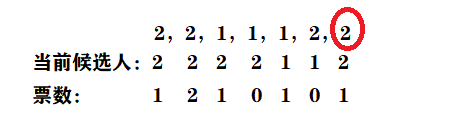# 多数元素int majorityElement1(vector<int>& nums)
{
sort(nums.begin(),nums.end());
return nums[nums.size()/2];
}


int majorityElement2(vector<int>& nums)
{
unordered_map<int,int> record;
for(int i=0;i<nums.size();i++)
{
record[nums[i]]++;
if(record[nums[i]]>nums.size()/2)
{
return nums[i];
}
}
return -1;
}int majorityElement (vector<int>& nums)
{
int cand=nums;
int count=1;
for(int i=1;i<nums.size();i++)
{
if(nums[i]==cand)
{
count++;
}
else if(--count<0)
{
cand=nums[i];
count=1;
}

}
return cand;

}©️2019 CSDN 皮肤主题: 大白 设计师: CSDN官方博客